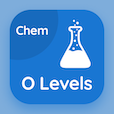Engineering Online Courses

Engineering Physics MCQs

Engineering Physics MCQ PDF - Topics

# Heat of Vaporization of Common Substances MCQ Quiz Online

Practice Heat of Vaporization of Common Substances Multiple Choice Questions (MCQ), Heat of Vaporization of Common Substances quiz answers PDF to learn engineering physics online course for engineering physics classes. First Law of Thermodynamics Multiple Choice Questions and Answers (MCQs), Heat of Vaporization of Common Substances quiz questions for free career test. "Heat of Vaporization of Common Substances MCQ" PDF Book: thermal expansion, absorption of heat by solids and liquids, heat of transformation, zeroth law of thermodynamics test prep for online high school college acceptance.

"Heat of vaporization of Mercury is" MCQ PDF: heat of vaporization of common substances with choices 296 kj/kg, 273 kj/ kg, 858 kj/ kg, and 2256 kj/ kg for free career test. Learn heat of vaporization of common substances quiz questions for merit scholarship test and certificate programs for associate degrees in engineering.

## MCQs on Heat of Vaporization of Common Substances Quiz

MCQ: Heat of vaporization of Mercury is

296 kJ/kg
273 kJ/ kg
858 kJ/ kg
2256 kJ/ kg

MCQ: 4730 kJ/Kg is the heat of vaporization of

Copper
Mercury
Silver

MCQ: Heat of vaporization of Water is

273 kJ/Kg
858 kJ/Kg
2256 kJ/Kg
2336 kJ/Kg

MCQ: 455 kJ/Kg is the heat of vaporization of

Silver
Copper
Water
Hydrogen

MCQ: Heat of vaporization of Oxygen is

296 kJ/Kg
213 kJ/Kg
2256 kJ/Kg
858 kJ/Kg

### More Topics from Engineering Physics Course